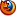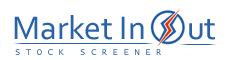Please enable JavaScript to view this page content properly Log In | Sign Up
 MA vs Price Crossovers EMA Crossovers WMA Crossovers Kaufman Adaptive MA MESA Adaptive MA Double EMA Triple EMA Wilder MA Volume Weighted MA Hull Moving Average
 MA vs Price Crossovers Action Price Crossed Above MA(7)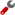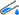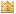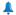Price Crossed Above MA(13)Price Crossed Above MA(26)Price Crossed Above MA(50)Price Crossed Above MA(200)Price Crossed Below MA(7)Price Crossed Below MA(13)Price Crossed Below MA(26)Price Crossed Below MA(50)Price Crossed Below MA(200)Price Above MA(7)Price Above MA(13)Price Above MA(26)Price Above MA(50)Price Above MA(200)Price Below MA(7)Price Below MA(13)Price Below MA(26)Price Below MA(50)Price Below MA(200)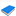Moving Averages - Technical Analysis from A to Z A Moving Average is an indicator that shows the average value of a security's price over a period of time. When calculating a moving average, a mathematical analysis of the security's average value over a predetermined time period is made. As the security's price changes, its average price moves up or down. The most popular method of interpreting a moving average is to compare the relationship between a moving average of the security's price with the security's price itself. A buy signal is generated when the security's price rises above its moving average and a sell signal is generated when the security's price falls below its moving average.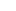Best viewed in